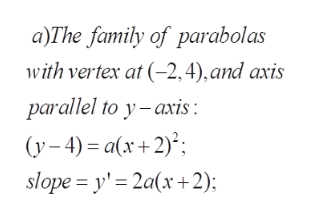# a. What is the family of orthogonal trajectories for the family of parabolas with axis parallel to the y-axis and vertex at the point (−2, 4)?b. Give the family of orthogonal trajectories of the family of parabolas with vertical axis and vertex at the point ( -6 , -4 ).

Question
45 views

a. What is the family of orthogonal trajectories for the family of parabolas with axis parallel to the y-axis and vertex at the point (−2, 4)?

b. Give the family of orthogonal trajectories of the family of parabolas with vertical axis and vertex at the point ( -6 , -4 ).

check_circle

Step 1

To determine the equations of the families of orthogonal trajectories for the given families of parabolas

Step 2

By definition, every member of the orthogonal family meets every member of the given family orthognally. That is, the tangents at the points of intersections are perpendicular.

Step 3help_outlineImage Transcriptionclosea)The family of parabolas with vertex at (-2,4), and axis parallel to y-axis: (y-4)a(x+2) slope = y' 2a(x+2) fullscreen

### Want to see the full answer?

See Solution

#### Want to see this answer and more?

Solutions are written by subject experts who are available 24/7. Questions are typically answered within 1 hour.*

See Solution
*Response times may vary by subject and question.
Tagged in

### Math